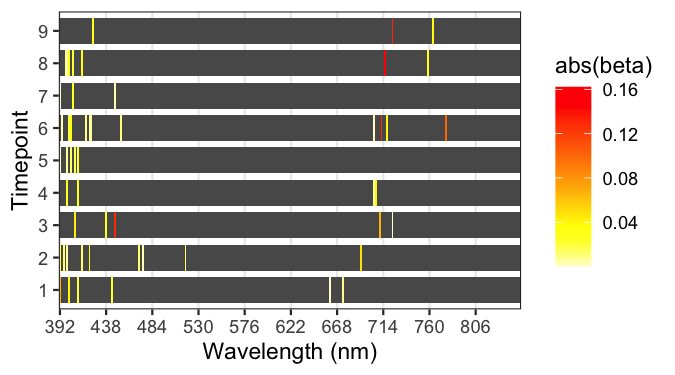# Standard Selection index

Recall the basic genetic model that decomposes phenotypic observations $$\boldsymbol{y}=(y_1,…,y_n)'$$, which are assumed to be centered and corrected by non-genetic effects (e.g., experiment and block effects), as the sum of breeding values, $$\boldsymbol{u}_y=(u_{y_1},…,u_{y_n})'$$, and environmental deviations, $$\boldsymbol{\varepsilon}_y=(\varepsilon_{y_1},…,\varepsilon_{y_n})'$$, as

$\begin{equation*} y_i=u_{y_i}+\varepsilon_{y_i} \end{equation*}$

Both $$\boldsymbol{u}_y$$ and $$\boldsymbol{\varepsilon}_y$$ are assumed to have null means and $$\text{var}(\boldsymbol{u}_y)=\sigma_{u_y}^2 \textbf{K}$$, $$\text{var}(\boldsymbol{\varepsilon}_y)=\sigma_{\varepsilon_y}^2 \textbf{I}$$, and $$\text{cov}(\boldsymbol{u},\boldsymbol{\varepsilon}')=\textbf{0}$$, where $$\sigma_{u_y}^2$$ and $$\sigma_{\varepsilon_y}^2$$ are the common genetic and residual variances, respectively; $$\textbf{K}$$ is the genetic relationship matrix and $$\textbf{I}$$ is an identity matrix.

A selection index (SI) (Hazel 1943; Smith 1936) is used to predict the genetic merit for a the selection goal ($$u_{y_i}$$, e.g., the genetic merit for grain yield of the $$i^\text{th}$$ genotype) as a linear combination of $$p$$ measured phenotypes, $$\boldsymbol{x}_i=(x_{i1},...,x_{ip})'$$, of the form

$\begin{equation*} \mathcal{I}_i=\boldsymbol{x}'_i\boldsymbol{\beta} \end{equation*}$

where $$\boldsymbol{\beta}=(\beta_1,...,\beta_p)'$$ is a vector of regression coefficients whose entries define the weights of each of the measured phenotypes in the SI.

In a standard selection index the weights are derived by minimizing the expected squared deviation between the genetic merit for the selection goal and the index, that is

$\begin{equation*} \hat{\boldsymbol{\beta}} = \underset{\beta}{\text{arg min}}\frac{1}{2}\mathbb{E}\left(u_{y_i}-\boldsymbol{x}'_i\boldsymbol{\beta}\right)^2 \end{equation*}$

Assuming that $$\boldsymbol{x}_i$$ follows also a genetic model (i.e., $$\boldsymbol{x}_i=\boldsymbol{u}_{x_i}+\boldsymbol{\varepsilon}_{x_i}$$) and that their environmental effects ($$\boldsymbol{\varepsilon}_{x_i}$$) are orthogonal to $$u_{y_i}$$, the above problem is equivalent to

$\begin{equation*} \hat{\boldsymbol{\beta}} = \underset{\beta}{\text{arg min}}\left[-\textbf{G}'_{x,y}\boldsymbol{\beta} + \frac{1}{2}\boldsymbol{\beta}'\textbf{P}_x\boldsymbol{\beta}\right] \end{equation*}$

where $$\textbf{G}_{x,y}=\mathbb{E}(u_{y_i}\boldsymbol{x}_i)$$ is a vector containing the genetic covariances between the selection target and each of the measured phenotypes, and $$\textbf{P}_x=\mathbb{E}(\boldsymbol{x}_i\boldsymbol{x}'_i)$$ is the phenotypic (co)variance matrix of $$\boldsymbol{x}_i$$. The solution to this optimization problem is

$\begin{equation*} \hat{\boldsymbol{\beta}} = \textbf{P}_x^{-1} \textbf{G}_{x,y} \end{equation*}$

The SI is by construction the best linear predictor (BLP) of the genetic merit for the selection goal, i.e., $$\mathbb{E}(u_{y_i}|\boldsymbol{x}_i)$$; this property holds when $$\textbf{G}_{x,y}$$ and $$\textbf{P}_x$$ are known.

# Regularized selection indices

## Principal components-based selection indices

Principal components (PC) can be obtained from the singular value decomposition of the real-valued matrix, $$\textbf{X}=[\boldsymbol{x}_1,\boldsymbol{x}_2,...,\boldsymbol{x}_n]'$$ (the matrix containing all measured traits) which takes the form $$\textbf{X}=\textbf{U}\textbf{D}\textbf{V}'$$. Matrix $$\textbf{U}=[\boldsymbol{u}_1,...,\boldsymbol{u}_p]$$ contains the left-singular vectors, $$\textbf{V}=[\boldsymbol{v}_1,...,\boldsymbol{v}_p]$$ is the matrix with the right-singular vectors, and $$\textbf{D}=\text{diag}(d_1,…,d_p)$$ is a diagonal matrix with positive or zero elements. The PCs $$\textbf{W}=\textbf{X}\textbf{V}=\textbf{U}\textbf{D}$$ are then linear combinations of the measured phenotypes. A principal components-based SI (PC-SI) uses the first $$q$$ PCs ($$\tilde{\textbf{W}}=[\boldsymbol{w}_1,...,\boldsymbol{w}_q]$$, $$q\leq p$$) as measured phenotypes in the SI, as

$\begin{equation*} \mathcal{I}_i=\tilde{\boldsymbol{w}}'_i\boldsymbol{\gamma}^{(q)} \end{equation*}$

where $$\tilde{\boldsymbol{w}}'_i$$ is a vector containing the scores for the $$i^{th}$$ observation on the first $$q$$ PCs and $$\boldsymbol{\gamma}^{(q)}$$ are their corresponding regression coefficients. The solution to the optimization problem takes the form $$\hat{\boldsymbol{\gamma}}^{(q)}=(n-1)(\tilde{\textbf{D}}^2)^{-1}\textbf{G}_{\tilde{w},y}$$, where $$\tilde{\textbf{D}}$$ contains only the first $$q$$ elements of $$\textbf{D}$$, and $$\textbf{G}_{\tilde{w},y}$$ is a vector containing the genetic covariances between each of the top $$q$$ PCs and the selection objective. This solution can be mapped to coefficients for the measured traits (as for the standard SI) using

$\begin{equation*} \hat{\boldsymbol{\beta}}^{(q)}=(n-1)\tilde{\textbf{V}}(\tilde{\textbf{D}}^2)^{-1}\textbf{G}_{\tilde{w},y} \end{equation*}$

where $$\tilde{\boldsymbol{V}}$$ is the matrix containing only the first $$q$$ right-singular vectors.

## Penalized selection indices

In a penalized regression, regularization is achieved by including in the objective function a penalty on model complexity. A penalized selection index (PSI) is obtained by considering an optimization problem as aforementioned, as follows:

$\begin{equation*} \hat{\boldsymbol{\beta}} = \underset{\beta}{\text{arg min}}\left[-\textbf{G}'_{x,y}\boldsymbol{\beta} + \frac{1}{2}\boldsymbol{\beta}'\textbf{P}_x\boldsymbol{\beta} + \lambda\cdot J(\boldsymbol{\beta})\right] \end{equation*}$

where $$\lambda$$ is a penalty parameter and $$J(\boldsymbol{\beta})$$ is a penalty function. Commonly used penalties include the L2 ($$||\boldsymbol{\beta}||^2_2=\sum_{j=1}^n\beta_{j}^2$$) and L1 ($$||\boldsymbol{\beta}||_1=\sum_{j=1}^n|\beta_{j}|$$) norms (Fu 1998) either alone or in a combination of both. We considered a penalty function as

$\begin{equation*} J(\boldsymbol{\beta})=\frac{1}{2} (1-\alpha) \sum_{j=1}^n\beta_j^2 + \alpha \sum_{j=1}^n|\beta_j| \end{equation*}$

where $$\alpha$$ is a weighting factor. This penalty is used in an regression (Zou and Hastie 2005) that combines the shrinkage-inducing feature of a ridge-regression (Hoerl and Kennard 1970), that uses the L2-norm alone, and the variable selection feature of a LASSO regression (Tibshirani 1996), that uses the L1-norm alone. With no penalization ($$\lambda=0$$), the solution for this penalized problem is equivalent to that of the standard SI.

The ridge-regression (L2-PSI) and LASSO (L1-PSI) types are special cases of the elastic-net-type PSI (EN-PSI) when $$\alpha=0$$ and $$\alpha=1$$, respectively. A closed-form solution for the regression coefficients can be found only for the L2-PSI (Hastie, Tibshirani, and Friedman 2009). In this case, the solution is

$\begin{equation*} \hat{\boldsymbol{\beta}}^{L2} = \left(\textbf{P}_x +\lambda \textbf{I}\right)^{-1} \textbf{G}_{x,y} \end{equation*}$

where $$\textbf{I}$$ is a $$p\times p$$ identity matrix. For the EN-PSI ($$0<\alpha\leq1$$) solutions are obtained using iterative algorithms such as least angle regression (LARS) (Efron et al. 2004) or coordinate descent (Friedman et al. 2007) for different values of the parameters $$\alpha$$ and $$\lambda$$. These combinations of the values of $$\alpha$$ and $$\lambda$$ will result in different EN-PSI from which an optimal index can be obtained such as the prediction accuracy is maximum.

# Phenotypic and genetic parameters

The population phenotypic (co)variance matrix $$\textbf{P}_x$$ among the measured phenotypes is estimated using the unbiased sample (co)variance matrix given by

$\begin{equation*} \hat{\textbf{P}}_x=\frac{1}{n-1}\sum_{i=1}^{n}\left(\boldsymbol{x}_i-\bar{\boldsymbol{x}}\right)\left(\boldsymbol{x}_i-\bar{\boldsymbol{x}}\right)' \end{equation*}$

where $$\bar{\boldsymbol{x}}$$ is the vector containing the sample mean of of the measured phenotypes. For centered data, this reduces to $$\hat{\textbf{P}}_x=\frac{1}{n-1}\textbf{X}'\textbf{X}$$, where $$\textbf{X} = [\boldsymbol{x}_1,\boldsymbol{x}_2,...,\boldsymbol{x}_n]'$$ is the matrix containing all measured traits.

The genetic covariance ($$\textbf{G}_{x_j,y}$$) between the target trait and the $$j^\text{th}$$ measured trait ($$j=1,...,p$$) is estimated using a sequence of uni-variate genetic models as previously mentioned. First, the model is fitted for the target trait as response, then for each of the measured traits, and then for the sum of the target trait and each of the measured traits. The genetic covariances between the target trait and the measured traits are then estimated using

$\begin{equation*} \hat{\textbf{G}}_{x_j,y}=\frac{1}{2}\left(\hat{\sigma}^2_{u_{y+xj}}-\hat{\sigma}^2_{u_y}-\hat{\sigma}^2_{u_{xj}}\right) \end{equation*}$

where $$\hat{\sigma}^2_{u_y}$$, $$\hat{\sigma}^2_{u_{xj}}$$ and $$\hat{\sigma}^2_{u_{y+xj}}$$ are the estimated genetic variances for the target trait, the $$j^\text{th}$$ measured trait, and the sum of the target trait and the $$j^\text{th}$$ measured trait, respectively.

# Accuracy of indirect selection

The accuracy of the index to be used for indirect selection, $$\text{Acc}(\mathcal{I})$$, is defined as the correlation between the index ($$\mathcal{I}_i$$) and the breeding values ($$u_i$$). This parameter is equal to the product of the square root of the heritability of the SI, $$h_\mathcal{I}$$, times the genetic correlation between the SI and the selection target, $$\text{cor}(u_{\mathcal{I}_i},u_{y_i})$$, this is

$\begin{equation*} \text{Acc}(\mathcal{I})=\text{cor}(\mathcal{I}_i,u_i)=\text{cor}(u_{\mathcal{I}_i},u_{y_i})h_\mathcal{I} \end{equation*}$

The efficiency of indirect selection relative to mass phenotypic selection ($$RE$$) is calculated as the ratio between the accuracy of indirect selection and the accuracy of direct selection, $$h_y$$, this is

$\begin{equation*} RE=\frac{h_\mathcal{I}}{h_y}\text{cor}(u_{\mathcal{I}_i},u_{y_i}) \end{equation*}$

where $$h_y$$ is the square root of the heritability of the selection goal ($$y$$), calculated as

$\begin{equation*} h_y^2=\frac{\sigma^2_{u_y}}{\sigma^2_{u_y} +\sigma^2_{e_y}} \end{equation*}$

# Cross validation

To avoid bias in the estimation, the accuracy of indirect selection must be estimated using data that was not used to derive the coefficients of the index. To this end, a training-testing partition procedure is implemented as follows: (i) data is partitioned into training (TRN) and testing (TST) sets, (ii) the coefficients of the SI, $$\boldsymbol{\beta}$$, are derived in the training set, (iii) these coefficients are applied to data of the testing set, $$\boldsymbol{x}_i$$, to derive an index ($$\mathcal{I}_i=\boldsymbol{x}'_i\boldsymbol{\beta}$$), and (iv) parameters $$\text{cor}(u_{\mathcal{I}_i},u_{y_i})$$, $$h_\mathcal{I}$$, and $$\text{Acc}(\mathcal{I})$$ are estimated in the testing set.

# Experimental data

The data set consists of 1,092 inbred wheat lines grouped into 39 trials and grown during the 2013- 2014 season at the Norman Borlaug experimental research station in Ciudad Obregon, Sonora, Mexico. Each trial consisted of 28 breeding lines that were arranged in an alpha-lattice design with three replicates and six sub-blocks. The trials were grown in four different environments:

Env Name Planting date Planting system Number of irrigations N genotypes (records)
E1 Flat-Drought Optimum Flat Minimal drip 1,092 (3,274)
E2 Bed-2IR Optimum Bed 2 1,090 (3,267)
E3 Bed-5IR Optimum Bed 5 1,092 (3,274)
E4 Bed-EHeat Early Bed 5 1,013 (2,858)

Records of grain yield (YLD, ton ha$$^{-1}$$) were collected as the total plot yield after maturity. Measurements for days to heading (DTH), days to maturity (DTM), and plant height (PH, ) were recorded only in the first replicate at each trial. Reflectance phenotypic data were collected from the fields using both infrared and hyper-spectral cameras mounted on an aircraft on 9 different dates (time-points) between January $$10^\text{th}$$ and March $$27^\text{th}$$, 2014. During each flight, data from 250 wavelengths ranging from 392 to 850 nm were collected for each pixel in the pictures. The average reflectance of all the pixels for each wavelength was calculated from each of the geo-referenced trial plots and reported as each line reflectance.

Grain yield and image data were pre-adjusted using mixed-effects model that accounted for genotype, trial, replicate, and sub-block. No pre-adjusting was made for DTH, DTM, and PH traits. Pre-adjusted phenotypes were obtained by subtracting from the phenotypic record the mean plus BLUPs of trial, replicate, and sub-block effects.

Lines were sequenced using GBS technology. Next, SNP were extracted and filtered so that lines >50% missing data were removed. Markers were recoded as –1, 0, and 1, corresponding to homozygous for the minor allele, heterozygous, and homozygous for the major allele, respectively. Next, markers with a minor allele frequency <0.05 and >15% of missing data were removed. Remaining SNPs with missing values were imputed with the mean of observed genotypes.

The CRAN version includes the wheatHTP dataset containing (un-replicated) YLD from all environments E1,…,E4, and reflectance (latest time-point only) data from the environment E1 only. Marker data is also included in the dataset. The phenotypic and reflectance data are averages (line effects from mixed models) for 776 lines evaluated in 28 trials (with at least 26 lines each) for which marker information on 3,438 SNPs is available.

The GitHub (development) version of the SFSI R-package (https://github.com/MarcooLopez/SFSI) includes also the wheatHTP dataset plus wheatHTP.E1,…,wheatHTP.E4 datasets containing replicated (adjusted) phenotypic and reflectance data for all four environments, respectively.

# Implementation

The above described data will be used to generate selection indices for grain yield (as selection target) using all the wavelengths (as measured phenotypes). Analyses will be implemented in R software (R Core Team 2019) using the SFSI R-package.

Data wil be taken from the SFSI R-package (GitHub version). Each file corresponds to one environment and contains objects Y, X, and VI. Matrix Y contains pre-adjusted observations for YLD, DTH, DTM, and PH. Object X is a list type containing the hyper-spectral image data for all 9 time-points. Element X[[j]], $$j=1,...,9$$, is a matrix with 250 columns (wavelengths) and rows matched with those of matrix Y. Likewise, the object VI is a list with 9 elements being two-columns matrices containing green and red NDVI. Following code shows how to prepare data for a single environment which will be used in later analyses. For demostration purposes, only data from 9 trials will be considered

rm(list = ls())

site <-  "https://github.com/MarcooLopez/SFSI/blob/master/data"
filename <- "wheatHTP.E3.RData"

trials <- as.numeric(as.character(Y$trial)) index <- trials %in% unique(trials)[1:9] # For ease, only 9 trials trials <- trials[index] Y <- Y[index,] X <- lapply(X,function(x)x[index,]) Y$gid <- factor(as.character(Y$gid)) Z <- model.matrix(~0+gid,data=Y) K <- tcrossprod(Z) # Connection of replicates y <- as.vector(Y[,"YLD"]) # Save file save(y,trials,K,X,file="prepared_data.RData") ## Heritability and variance components Heritability can be calculated from variance components obtained by fitting the aforementioned genetic model for the whole data. Code below illustrates how to fit this model using the function fitBLUP from the SFSI R-package. library(SFSI) # Load data load("prepared_data.RData") evdK <- eigen(K) # Decomposition of K # Fit model y <- as.vector(scale(y)) fm0 <- fitBLUP(y,U=evdK$vectors,d=evdK$values,BLUP=FALSE) c(fm0$varU,fm0$varE,fm0$h2)

save(fm0,evdK,file="varComps.RData")

## Training-testing partitions

Code below illustrates how to split data into many TRN and TST sets. In this example, $$\frac{2}{3}$$ of the 9 trials (6 trials, $$n_{TRN}\approx 504$$ observations) will be randomly assigned to the training set and the remaining $$\frac{1}{3}$$ (3 trials, $$n_{TST}\approx 252$$) to the testing set. The output will be a matrix whose columns are vectors with 1’s and 2’s indicating which observations are assigned to the training and testing sets, respectively.

The time-point to be analyzed can be specified through variable timepoints thus it can be set to calculate covariances for all the 9 time-points. The number of TRN-TST partitions to run are specified through parameter nPart

The paramaters will be saved in the file paramaters.RData and will be used later for single time-point and across time-points analyses.

load("prepared_data.RData") # load data

#---------- parameters ------------#
pTST <- 0.3           # Perc of the trials to assign to testing
nPart <- 5            # Number of TRN-TST partitions to perform
timepoints <- 6:9     # Time-points to analyze
#----------------------------------#

nTrial <- length(unique(trials))
nTST <- ceiling(pTST*nTrial)  # Number of trials in TST set

seeds <- round(seq(1E3, .Machine$integer.max, length = 500)) partitions <- matrix(1,nrow=length(y),ncol=length(seeds)) # Object to store partitions for(k in 1:length(seeds)) { set.seed(seeds[k]) tst <- sample(unique(trials),nTST,replace=FALSE) partitions[which(trials %in% tst),k] <- 2 } save(partitions,pTST,nPart,timepoints,file="parameters.RData") ## Genetic covariances estimation The genetic covariances between the grain yield and each of the wavelengths will be calculated using a sequence of genetic models implemented in the function getGenCov from the SFSI package. Code in snippet below shows how to calculate genetic and phenotypic covariances within time-point. Calculations are performed for each partition using data from training set only. Results are stored in matrices gencov and phencov, and saved in the file covariances_tp_*.RData. load("prepared_data.RData"); load("parameters.RData") for(tp in timepoints){ gencov <- phencov <- c() # Matrices to store covariances for(k in 1:nPart) { cat(" partition = ",k,"of",nPart,"\n") indexTRN <- which(partitions[,k]==1) # Training set xTRN <- scale(X[[tp]][indexTRN,]) yTRN <- as.vector(scale(y[indexTRN])) KTRN <- K[indexTRN,indexTRN] # Relationship matrix (given by replicates) # Get genetic variances and covariances fm <- getGenCov(y1=yTRN,y2=xTRN,K=KTRN,scale=FALSE,warn=FALSE,mc.cores=1) gencov <- cbind(gencov,fm$covU)
phencov <- cbind(phencov,fm$covU + fm$covE)
}
save(gencov,phencov,file=paste0("covariances_tp_",tp,".RData"))
cat("Time-point=",tp,". Done \n")
}

## Single time-point selection indices

Standard, L1-penalized and PC-based SIs will be fitted using data from a single time-point. The regression coefficients are to be obtained from training data within each partition previously created.

### Regression coefficients

Following code can be used to estimate regression coefficients using training data within each partition for the standard SI and for the PC-SI (with $$1, 2,...,250$$ PCs). Coefficients for L1-PSI are estimated the whose solutions are found using either the functions LARS or solveEN from the SFSI R-package. Function solveEN will yield solutions for a given grid of values of $$\lambda$$ while function LARS provides solutions for the entire $$\lambda$$ path.

Time-point can be specified through variable timepoints. Regression coefficients are stored in matrices bSI, bPCSI and bL1PSI, and saved in the file coefficients_tp_*.RData. Calculations are performed using genetic covariances calculated for the partitions previously created.

load("prepared_data.RData"); load("parameters.RData")

for(tp in timepoints){

# Objects to store regression coefficients
bSI <- bPCSI <- bL1PSI <- vector("list",nPart)

for(k in 1:nPart)
{ cat("  partition = ",k,"of",nPart,"\n")
indexTRN <- which(partitions[,k]==1)

# Training set
xTRN <- scale(X[[tp]][indexTRN,])
VARx <- var(xTRN)
EVDx <- eigen(VARx)

# Standard SI
VARinv <- EVDx$vectors %*% diag(1/EVDx$values) %*% t(EVDx$vectors) bSI[[k]] <- VARinv %*% gencov[,k] # PC-based SI VARinv <- diag(1/EVDx$values)
gamma <- as.vector(VARinv %*% t(EVDx$vectors) %*% gencov[,k]) beta <- apply(EVDx$vectors %*% diag(gamma),1,cumsum)
bPCSI[[k]] <- data.frame(I(beta),df=1:nrow(beta),lambda=NA)

# L1-PSI
fm <- solveEN(VARx, gencov[,k],nlambda=100)
# fm <- LARS(VARx, gencov[,k])  # Second option
beta <- t(fm$beta)[-1,] bL1PSI[[k]] <- data.frame(I(beta),df=fm$df[-1],lambda=fm$lambda[-1]) } save(bSI,bPCSI,bL1PSI,file=paste0("coefficients_tp_",tp,".RData")) cat("Time-point=",tp,". Done \n") } ### Accuracy of the index The regression coefficients above calculated can be applied to data from testing set to derive selection indices ($$\mathcal{I}_i$$). Code below can be used to (i) compute the SIs for the testing data, and then (ii) calculate the accuracy of the index, the heritability of the index, and genetic correlation of the index with grain yield. These (co)variance components are obtained using the function getGenCov. Results for the three indices (standard SI, PC-SI and L1-PSI) are stored in the object matrix accSI and saved in the file accuracy_tp_*.RData. Calculations are performed across all partitions previously created. load("prepared_data.RData"); load("parameters.RData") for(tp in timepoints){ load(paste0("coefficients_tp_",tp,".RData")) accSI <- c() # Object to store accuracy components for(k in 1:nPart) { cat(" partition = ",k,"of",nPart,"\n") indexTST <- which(partitions[,k]==2) # Testing set xTST <- scale(X[[tp]][indexTST,]) yTST <- as.vector(scale(y[indexTST])) KTST <- K[indexTST,indexTST] # Connection given by replicates # Calculate the indices SI <- xTST %*% bSI[[k]] PCSI <- tcrossprod(xTST, bPCSI[[k]]$beta)
L1PSI <- tcrossprod(xTST, bL1PSI[[k]]$beta) # Fit genetic models fitSI <- as.matrix(data.frame(I(SI),I(PCSI),I(L1PSI))) fm <- getGenCov(y1=yTST,y2=fitSI,K=KTST,warn=FALSE,mc.cores=1) fm$varU2 <- ifelse(fm$varU2<0.05,0.05,fm$varU2)

# Retrieve accuracy components
h <- sqrt(fm$varU2/(fm$varU2 + fm$varE2)) gencor <- fm$covU/sqrt(fm$varU1*fm$varU2)
acc2 <- fm$covU/sqrt(fm$varU1)
accuracy <- abs(gencor)*h

df <- c(nrow(bSI[[k]]),bPCSI[[k]]$df,bL1PSI[[k]]$df)
lambda <- c(min(bL1PSI[[k]]$lambda),bPCSI[[k]]$lambda,bL1PSI[[k]]$lambda) accSI <- rbind(accSI,data.frame(rep=k,SI=colnames(fitSI),h,gencor,accuracy,df,lambda)) } save(accSI,file=paste0("accuracy_tp_",tp,".RData")) cat("Time-point=",tp,". Done \n") } ### Effect of regularization on the accuracy of the index The regularized selection indices were calculated for $$q=1,2,...,250$$ PCs for the PC-SI and for decreasing values, $$\lambda_1,...,\lambda_{100}$$, of the path of the penalization for the L1-PSI. In the later case, decreasing $$\lambda$$ will give increasing number of active number of predictors in the index. Code in the following snippet shows how to create a plot depicting the evolution of accuracy (and its components) over values of regularization (number of PCs or number of active predictors). Results for the standard SI corresponds to the case of a PC-SI with 250 PCs and a L1-PSI with 250 active predictors. These cases are shown at the right-most results in the plots. tp <- 9 # Time-point load(paste0("accuracy_tp_",tp,".RData")) accSI = split(accSI,as.character(accSI$SI))
accSI=data.frame(do.call(rbind,lapply(accSI,function(x)apply(x[,-c(1,2)],2,mean,na.rm=T))))
accSI$SI = unlist(lapply(strsplit(rownames(accSI),"\\."),function(x)x)) # Plot of PC-SI if(requireNamespace("reshape") & requireNamespace("ggplot2")){ dat <- reshape::melt(accSI[accSI$SI=="PCSI",],id=c("df","lambda","SI"))
plot1 <- ggplot2::ggplot(dat,ggplot2::aes(df,value,group=variable,color=variable)) +
ggplot2::theme_bw() + ggplot2::geom_line(size=1) +
ggplot2::labs(title="PC-SI",y="correlation",x="Number of PCs")

# Plot of L1-PSI
dat <- reshape::melt(accSI[accSI$SI=="L1PSI",],id=c("df","lambda","SI")) plot2 <- ggplot2::ggplot(dat,ggplot2::aes(df,value,group=variable,color=variable)) + ggplot2::theme_bw() + ggplot2::geom_line(size=1) + ggplot2::labs(title="L1-PSI",y="correlation",x="Number of active predictors") plot1; plot2 }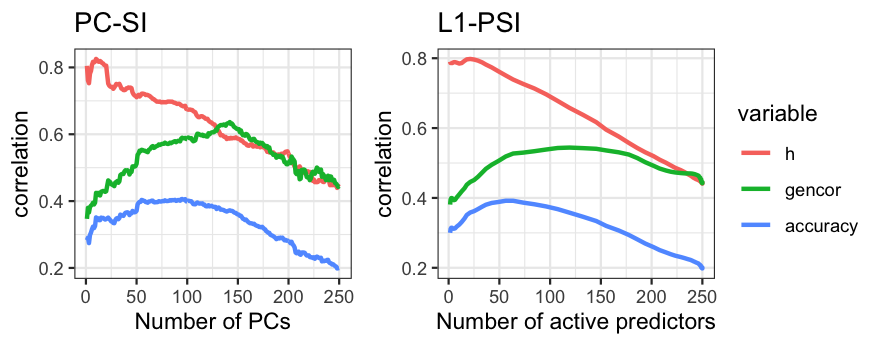### Optimal regularized vs standard selection indices An optimal regularized selection index can be chosen by selecting an optimal regularization (number of PCs or value of $$\lambda$$) such that the accuracy of selection of the resulting index in the testing set is maximum. Code below can be used to select the optimal PC-SI and L1-PSI, and to compare the accuracy of selection with that of the standard SI (non-regularized). Results are reported as an average across all partitions along with a $$95\%$$ confidence interval. tp <- 9 # Time-point load(paste0("accuracy_tp_",tp,".RData")) accSI$Model <- unlist(lapply(strsplit(as.character(accSI$SI),"\\."),function(x)x)) accSI <- split(accSI,paste(accSI$rep,"_",accSI$Model)) accSI <- do.call(rbind,lapply(accSI,function(x)x[which.max(x$accuracy),]))

dat = aggregate(accuracy ~ Model, mean, data=accSI)
dat$sd = aggregate(accuracy ~ Model,sd,data=accSI)$accuracy
dat$n = aggregate(accuracy ~ Model,length,data=accSI)$accuracy
dat$se = qnorm(0.975)*dat$sd/sqrt(dat$n) if(requireNamespace("ggplot2")){ ggplot2::ggplot(dat,ggplot2::aes(Model,accuracy)) + ggplot2::theme_bw() + ggplot2::geom_bar(stat="identity",width=0.6,fill="orange") + ggplot2::geom_errorbar(ggplot2::aes(ymin=accuracy-se,ymax=accuracy+se),width=0.2) + ggplot2::geom_text(ggplot2::aes(label=sprintf("%.2f",accuracy),y=accuracy*0.5)) }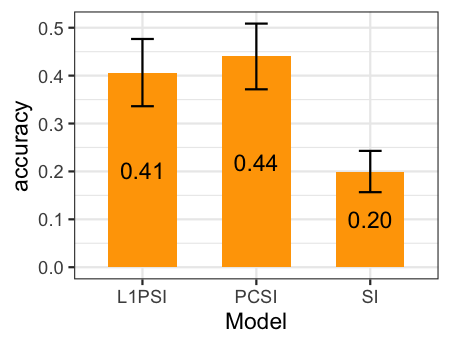### Comparison of the indices across time-points The accuracy of indirect selection for the optimal (i.e., the one with the highest accuracy of indirect selection) L1-PSI and PC-SI can be assessed across time-points and compared with a standard SI. Code in box below can be used to calculate the optimal PC-SI and L1-PSI, and to compare their accuracy of selection with that of the standard SI across all time-points. To this end, all the within time-point analyses (calculation of genetic covariances, regression coefficients, and prediction accuracy) must be previously performed for all the 9 time-points. This can be achieved using, for instance, by setting: timepoints = 1:9. load("parameters.RData") AccSI <- c() for(tp in timepoints) { load(paste0("accuracy_tp_",tp,".RData")) AccSI <- rbind(AccSI,data.frame(Timepoint=tp,accSI)) } AccSI$Model <- unlist(lapply(strsplit(as.character(AccSI$SI),"\\."),function(x)x)) AccSI <- split(AccSI,paste(AccSI$Timepoint,"_",AccSI$rep,"_",AccSI$Model))
AccSI <- do.call(rbind,lapply(AccSI,function(x)x[which.max(x$accuracy),])) AccSI$Timepoint <- factor(as.character(AccSI$Timepoint)) dat = aggregate(accuracy ~ Model+Timepoint,mean,data=AccSI) dat$sd = aggregate(accuracy ~ Model+Timepoint,sd,data=AccSI)$accuracy dat$n = aggregate(accuracy ~ Model+Timepoint,length,data=AccSI)$accuracy dat$se = qnorm(0.975)*dat$sd/sqrt(dat$n)

if(requireNamespace("ggplot2")){
ggplot2::ggplot(dat,ggplot2::aes(Timepoint,accuracy,color=Model,group=Model)) +
ggplot2::theme_bw() + ggplot2::geom_line() + ggplot2::geom_point() #+ geom_errorbar(aes(ymin=accuracy-se,ymax=accuracy+se),width=0.2)
}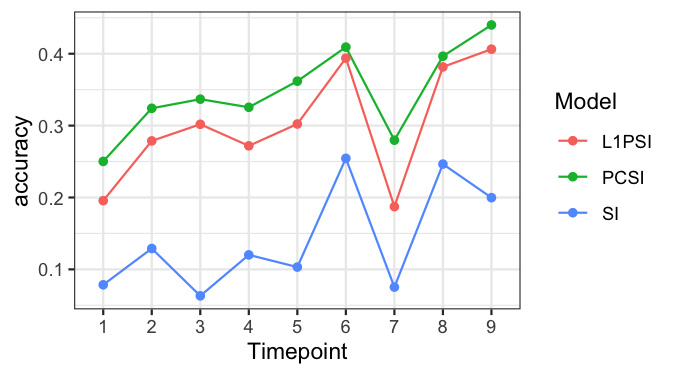## Multi time-point selection indices

Selection indices can be calculated to include reflectance data from multiple time-points. In this case, the vector of measured phenotypes $$\boldsymbol{x}_i$$ will contain $$2,250$$ traits corresponding to 250 wavebands measured at each of 9 time-points.

### Regression coefficients

Code in box below shows how to calculate coefficients for the PC-SI and L1-PSI using data from all 9 time-points. To this end, the genetic covariances of all $$2,250$$ traits with grain yield are needed, thus, all the within time-point genetic covariances must be previously calculated for all the 9 time-points. Results are saved in file multi_timepoint_coefficients.RData.

PC-SIs will be calculated including $$1,2, ...,500$$ PCs only (the maximum possible is $$2,250$$ PCs). The function solveEN will be used to calculate the regression coefficients for the L1-PSI using a grid of 100 values of $$\lambda$$.

load("prepared_data.RData"); load("parameters.RData")

Gencov <- c()   # To stack all covariances from all time-points
for(tp in timepoints)
Gencov <- rbind(Gencov,gencov)
}

# Objects to store regression coefficients
bPCSI <- bL1PSI <- vector("list",nPart)

for(k in 1:nPart)
{   cat("  partition = ",k,"of",nPart,"\n")
indexTRN <- which(partitions[,k]==1)

# Training set
xTRN <- scale(do.call(cbind,X[timepoints])[indexTRN,])
VARx <- var(xTRN)
EVDx <- eigen(VARx)

# PC-based SI
gamma <- t(sweep(EVDx$vectors,2,1/EVDx$values,FUN="*")) %*%  Gencov[,k]
beta <- apply(sweep(EVDx$vectors,2,as.vector(gamma),FUN="*"),1,cumsum)[1:500,] bPCSI[[k]] <- data.frame(I(beta),df=1:nrow(beta),lambda=NA) # L1-PSI fm <- solveEN(VARx, Gencov[,k],nlambda=100,maxiter=200,tol=1E-3) beta <- t(fm$beta)[-1,]
bL1PSI[[k]] <- data.frame(I(beta),df=fm$df[-1],lambda=fm$lambda[-1])
}
save(bPCSI,bL1PSI,nPart,file="multi_timepoint_coefficients.RData")

### Accuracy of the index

As for the single time-point, the regression coefficients are applied to data from testing set to derive selection indices. The following code shows how to (i) compute the multi time-point PC-SI and L1-PSI for the testing data, and then (ii) calculate the accuracy of the index, the heritability of the index, and genetic correlation of the index with grain yield. The genetic covariances are obtained using the function getGenCov.

Results for the two indices (PC-SI and L1-PSI) are stored in the object matrix AccSI and saved in the file multi_timepoint_accuracy.RData. Calculations are performed across all partitions previously created.

load("parameters.RData")

AccSI <- c()     # Objects to store accuracy components

for(k in 1:nPart)
{   cat("  partition = ",k,"of",nPart,"\n")
indexTST <- which(partitions[,k]==2)

# Testing set
xTST <- scale(do.call(cbind,X[timepoints])[indexTST,])
yTST <- as.vector(scale(y[indexTST]))
KTST <- K[indexTST,indexTST]   # Connection given by replicates

# Calculate the indices
PCSI_multi <- tcrossprod(xTST, bPCSI[[k]]$beta) L1PSI_multi <- tcrossprod(xTST, bL1PSI[[k]]$beta)

# Fit genetic models
fitSI <- as.matrix(data.frame(I(PCSI_multi),I(L1PSI_multi)))
fm <- getGenCov(y1=yTST,y2=fitSI,K=KTST,warn=FALSE,mc.cores=1)
fm$varU2 <- ifelse(fm$varU2<0.05,0.05,fm$varU2) # Retrieve accuracy components h <- sqrt(fm$varU2/(fm$varU2 + fm$varE2))
gencor <- fm$covU/sqrt(fm$varU1*fm$varU2) accuracy <- gencor*h df <- c(bPCSI[[k]]$df,bL1PSI[[k]]$df) lambda <- c(bPCSI[[k]]$lambda,bL1PSI[[k]]$lambda) AccSI <- rbind(AccSI,data.frame(rep=k,SI=colnames(fitSI),h,gencor,accuracy,df,lambda)) } save(AccSI,file="multi_timepoint_accuracy.RData") ### Optimal multi vs single time-point selection indices As for the single time-point case, one can obtain an optimal regularized selection index. Code in the following box can be used to select the optimal PC-SI and L1-PSI, and to compare the accuracy of selection with that of a single time-point (specified by parameter tp) standard SI, PC-SI and L1-PSI. Results are reported as an average across all partitions along with a $$95\%$$ confidence interval. tp <- 9 # Time-point load(paste0("accuracy_tp_",tp,".RData")) load("multi_timepoint_accuracy.RData") AccSI <- rbind(accSI,AccSI) AccSI$Model <- unlist(lapply(strsplit(as.character(AccSI$SI),"\\."),function(x)x)) AccSI <- split(AccSI,paste(AccSI$rep,"_",AccSI$Model)) AccSI <- do.call(rbind,lapply(AccSI,function(x)x[which.max(x$accuracy),]))

dat = aggregate(accuracy ~ Model, mean,data=AccSI)
dat$sd = aggregate(accuracy ~ Model, sd, data=AccSI)$accuracy
dat$n = aggregate(accuracy ~ Model, length, data=AccSI)$accuracy
dat$se = qnorm(0.975)*dat$sd/sqrt(dat$n) if(requireNamespace("ggplot2")){ ggplot2::ggplot(dat,ggplot2::aes(Model,accuracy)) + ggplot2::theme_bw() + ggplot2::geom_bar(stat="identity",width=0.65,fill="orange") + ggplot2::geom_errorbar(ggplot2::aes(ymin=accuracy-se,ymax=accuracy+se),width=0.2) + ggplot2::geom_text(ggplot2::aes(label=sprintf("%.2f",accuracy),y=accuracy*0.5)) }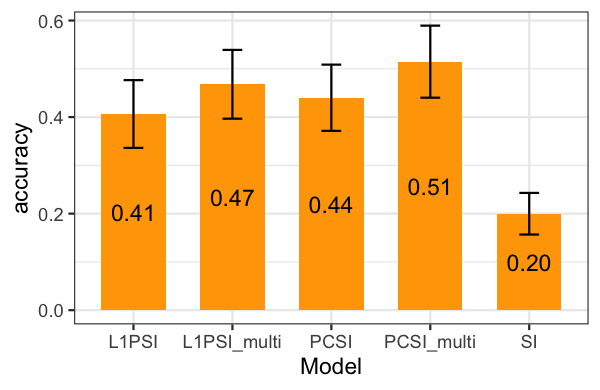## Sparsity of the index The value of the penalization parameter $$\lambda$$ in the L1-PSI define the number of predictors with non-zero coefficient in the index. Code below can be used to estimate the regression coefficients for a multi time-point L1-PSI for the whole dataset. To achieve this, the genetic covariances will be calculated for the $$2,250$$ wavelengths using whole data by means of the function getGenCov. The coefficients are then obtained using the function solveEN with a value of $$\lambda$$ obtained from the partitions. A heatmap of the coefficients will be generated to show the sparsity and regions of the electromagnetic spectrum that were selected by the method. load("varComps.RData") # Load the SVD of ZZ' to speed computation load("multi_timepoint_accuracy.RData") # Get genetic covariances x <- scale(do.call(cbind,X)) fm <- getGenCov(y1=y,y2=x,U=evdK$vectors,d=evdK$values, mc.cores=5,warn=FALSE) gencov <- fm$covU

# Get a value of lambda across partitions
AccSI$Model <- unlist(lapply(strsplit(as.character(AccSI$SI),"\\."),function(x)x))
AccSI <- split(AccSI,paste0(AccSI$Model,"_",AccSI$rep))
AccSI <- do.call(rbind,lapply(AccSI,function(x)x[which.max(x$accuracy),])) lambda <- mean(AccSI$lambda[AccSI$Model=="L1PSI_multi"]) # Get regression coefficients VARx <- var(x) beta <- solveEN(VARx,gencov,lambda=lambda)$beta

wl <- factor(rep(gsub("wl","",colnames(X[])),length(X)))
Timepoint <- factor(rep(seq(length(X)),each=ncol(X[])))
dat <- data.frame(beta=as.vector(beta),Timepoint,wl)
dat$beta[abs(dat$beta) < .Machine\$double.eps] <- NA

if(requireNamespace("ggplot2")){
ggplot2::ggplot(dat, ggplot2::aes(x=wl,y=Timepoint,fill = abs(beta))) +
ggplot2::theme_bw() + ggplot2::geom_tile(height=0.8) + ggplot2::labs(x="Wavelength (nm)") +
ggplot2::scale_x_discrete(breaks=levels(wl)[seq(1,nlevels(wl),25)]) +
}